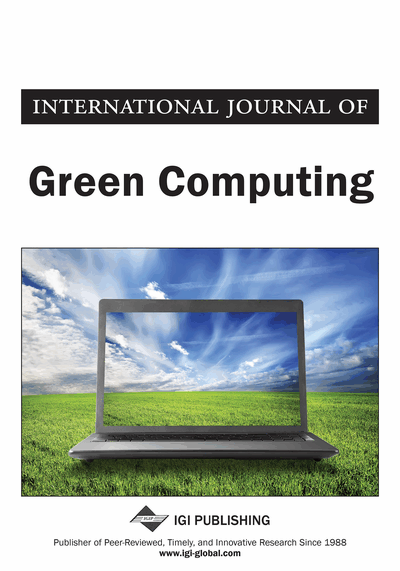# An Alternative Approach for Evaluating the Binormal ROC Curve

R. Amala (Department of Statistics, Ramanujan School of Mathematical Sciences, Pondicherry University, Puducherry, India) and R. Vishnu Vardhan (Department of Statistics, Ramanujan School of Mathematical Sciences, Pondicherry University, Puducherry, India)
DOI: 10.4018/jgc.2013010101
Available
\$37.50
No Current Special Offers

## Abstract

In recent years the ROC curve analysis has got its attention in almost all diversified fields. Basing on the data pattern and its distribution various forms of ROC models have been derived. In this paper, the authors have assumed that the data of two populations (healthy and diseased) follows normal distribution, it is one of the most commonly used forms under parametric approach. The present paper focuses on providing an alternative approach for the tradeoff plot of ROC curve and the computation of AUC using a special function of sigmoid shape called Error function. It is assumed that the test scores of particular biomarker are normally distributed. The entire work has been carried out for providing a new approach for the construction of Binormal ROC curve, which makes use of Error function which can be called as ErROC curve. The summary measure AUC of the resulting ErROC curve has been estimated and defined as ErAUC. The authors have also focused on deriving the expression for obtaining the optimal cut-off point. The new ErROC curve model will provide the true positive rate value at each and every point of false positive rate unlike conventional Binormal ROC model.
Article Preview
Top

## Introduction

In every circumstances of life we come across the problem of classifying an object or individuals into a desirable group based on some inherent characteristics of the object or individual. Unfortunately, the classification made is not perfect or the objects may be misclassified. In order to quantify the classifier performance, Receiver Operating Characteristic (ROC) curves has long been used as a major tool since 1950. It has been used for detecting the radar signals. From 1960, it has been extensively used by the radiologist and clinicians for classifying the individual into diseased or healthy (Swets, 1979). Since then, lot of development has been made in ROC curve analysis in three different approaches like parametric, non-parametric and semi-parametric.

In parametric approach, much work has been done over the years. This approach constitutes robust mathematical structures, where these help in evaluating the performance of a diagnostic test and also enables to assess the effect of covariates in the model. We reviewed some of the recent contributions in this approach. It is noticed that, during a decade of time, many researchers have contributed much in developing the ROC curve model by incorporating the covariate effects, different estimating procedures etc.

Ugwu et al. (2010) explored the potency of machine learning technique (decision tree) in development of a malaria diagnostic system. The decision tree algorithm was used in the development of the knowledge base. During the diagnosis, symptoms are provided by the patient in the diagnostic system and a match is found in the knowledge base.

James A. Hanley (1988) has proved the robustness of using the bi-normal model to fit the ROC curves.Pepe M.S. (2000) has proposed a General linear model approach for evaluating the ROC form and as well as estimating the AUC. A linear regression frame work (Zang Zheng, Pepe, M.S. (2005) has been introduced to evaluate the ROC model by involving the covariate effects on the ROC curves. Fawcett Tom et al., (2007) studied the non-convex ROC curves and the conditions that can lead to empirical and fitted ROC curves that are not convex.

Metz, C.E. (2010) has proposed a proper bi-normal ROC curve for improving the accuracy. The terminology used for proper bi-normal ROC curve is the ‘hook’. This means that the new defined ROC curve will get boosted at the curvilinear part and provides better accuracy when compared to the conventional form of Binormal ROC curve. Ethan J, et al. (2010) have projected the Comparison of ROC curves on the basis of optimal operating point. Davidov, O., & Nov, Y. (2011) proposed an improved, consistent and asymptotically normally distributed estimator which outperforms the original estimator of Hsieh and Turnball in 1996.

Hanley and McNeil (1982) have proposed a method for comparing the area under two ROC curves. Halpern, Schisterman, Enrique F et al. (2010) estimated the ROC curve and gave an approximation for the confidence interval of the linear combination of multiple bio-markers, by assuming that the covariates are adjusted and follow multivariate normal distribution.

Hsieh and Turnbull (1996) have proposed non-parametric methods for evaluating diagnostic tests. Farragi and Reiser (2002) have proved that the bi-normal model performs very similar to the non-parametric approach by simulation studies.

In semi-parametric approach, Tianxi Cai and Chaya S. Moskowitz, 2004 have proposed two new methods such as pseudo-maximum likelihood method and profile likelihood for estimating the parameters of bi-normal model. Zhou and Lin (2007) have proposed a new semi-parametric maximum likelihood estimate of an ROC curve that satisfies the property of invariance of the ROC curve.

Zhenqiu, L. and Ming, T. (2008) have proposed a nonparametric method to directly maximize the weighted specificity and sensitivity of the receiver operating characteristic curve. And the proposed method assigns different error costs to different classes explicitly.

Andriy, I.B., Howard, E.R., Tao, S. and David, G. (2009) have proposed a new index of the free-response performance at all decision thresholds simultaneously and this can be regarded as an area under the empirical free response ROC curve . They have also developed a non-parametric method for its analysis. They have also derived the ideal bootstrap estimator of the variance.

## Complete Article List

Search this Journal:
Reset
Open Access Articles: Forthcoming
Volume 10: 1 Issue (2019)
Volume 9: 2 Issues (2018)
Volume 8: 2 Issues (2017)
Volume 7: 1 Issue (2016)
Volume 6: 2 Issues (2015)
Volume 5: 2 Issues (2014)
Volume 4: 2 Issues (2013)
Volume 3: 2 Issues (2012)
Volume 2: 2 Issues (2011)
Volume 1: 2 Issues (2010)
View Complete Journal Contents Listing